# Feedback Amplifier Design

This example shows the design of a non-inverting feedback amplifier circuit using Control System Toolbox™. This design is built around the operational amplifier (op amp), a standard building block of electrical feedback circuits.

This tutorial shows how a real electrical system can be designed, modeled, and analyzed using the tools provided by Control System Toolbox.

### Op Amp Description

The standard building block of electrical feedback circuits is the operational amplifier (op amp), a differential voltage amplifier designed to have extremely high dc gain, often in the range of 1e5 to 1e7.

The electrical symbol for the op amp is shown below.This example assumes the use of an uncompensated op amp with 2 poles (at frequencies w1,w2) and high dc gain (a0). Assuming this op amp is operated in its linear mode (not saturated), then its open-loop transfer function can be represented as a linear time-invariant (LTI) system, as shown above.

Though higher-order poles will exist in a physical op amp, it has been assumed in this case that these poles lie in a frequency range where the magnitude has dropped well below unity.

Open-Loop Transfer Function:The following system parameters are assumed:a0 = 1e5; w1 = 1e4; w2 = 1e6; 

Next, you want to create a transfer function model of this system using Control System Toolbox. This model will be stored in the MATLAB® workspace as an LTI object.

First, define the Laplace variable, s, using the TF command. Then use 's' to construct the open-loop transfer function, a(s):

s = tf('s'); a = a0/(1+s/w1)/(1+s/w2) 
a = 1e15 ---------------------- s^2 + 1.01e06 s + 1e10 Continuous-time transfer function. 

You can view the frequency response of a(s) using the BODEPLOT command:

h = bodeplot(a,'r'); setoptions(h,'FreqUnits','rad/s','MagUnits','dB','PhaseUnits','deg',... 'YLimMode','Manual','YLim',{[0,110],[-180,0]});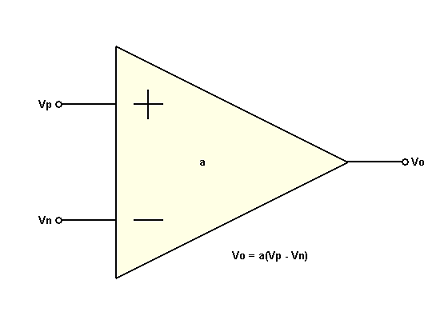Right-click on the plot to access a menu of properties for this Bode Diagram. Left-click on the curves to create moveable data markers which can be used to obtain response details.

You can view the normalized step response of a(s) using the STEPPLOT and DCGAIN commands:

a_norm = a / dcgain(a); stepplot(a_norm,'r') title('Normalized Open-Loop Step Response'); ylabel('Normalized Amplitude');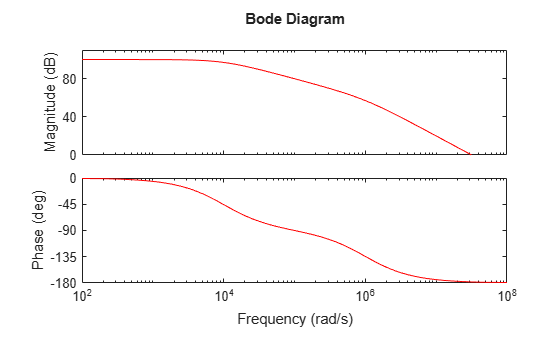Right-click on the plot and select "Characteristics -> Settling Time" to display the settling time. Hold the mouse over the settling time marker to reveal the exact value of the settling time.

### Feedback Amplifier

Now add a resistive feedback network and wire the system as a non-inverting amplifier.This feedback network, b(s), is simply a voltage divider with input Vo and output Vn. Solving for the ratio Vn/Vo yields the transfer function for b(s):

 b = Vn / Vo = R1 / (R1 + R2)

The block diagram representation of the system is shown below.Solving for the ratio Vo/Vp yields the closed-loop gain, A(s):

 A = Vo / Vp = a / (1 + ab)

If the product 'ab' is sufficiently large (>>1), then A(s) may be approximated as

 A = 1 / b

Now assume that you need to design an amplifier of dc gain (Vo/Vp) 10 and that R1 is fixed at 10 kOhm. Solving for R2 yields:

A0 = 10; b = 1 / A0; % approximation for ab>>1 R1 = 10000; R2 = R1 * (1/b - 1) 
R2 = 90000 

Construct the closed-loop system using the FEEDBACK command:

A = feedback(a,b); 

Next, plot the frequency responses of a(s) and A(s) together using the BODEMAG command:

bodemag(a,'r',A,'b'); legend('Open-Loop Gain (a)','Closed-Loop Gain (A)') ylim([0,110]); % Annotations opampdemo_annotate(1)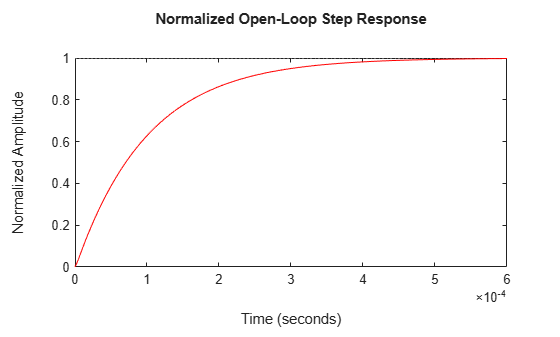The use of negative feedback to reduce the low-frequency (LF) gain has led to a corresponding increase in the system bandwidth (defined as the frequency where the gain drops 3dB below its maximum value).

This gain / bandwidth tradeoff is a powerful tool in the design of feedback amplifier circuits.

Since the gain is now dominated by the feedback network, a useful relationship to consider is the sensitivity of this gain to variation in the op amp's natural (open-loop) gain.

Before deriving the system sensitivity, however, it is useful to define the loop gain, L(s)=a(s)b(s), which is the total gain a signal experiences traveling around the loop:

L = a * b; 

You will use this quantity to evaluate the system sensitivity and stability margins.

The system sensitivity, S(s), represents the sensitivity of A(s) to variation in a(s).The inverse relationship between S(s) and L(s) reveals another benefit of negative feedback: "gain desensitivity".

S = 1 / (1 + L); 

S(s) has the same form as the feedback equation and, therefore, may be constructed using the more-robust FEEDBACK command:

S = feedback(1,L); 

The magnitudes of S(s) and A(s) may be plotted together using the BODEMAG command:

bodemag(A,'b',S,'g') legend('Closed-Loop Gain(A)', 'System Sensitivity(S)','Location','SouthEast')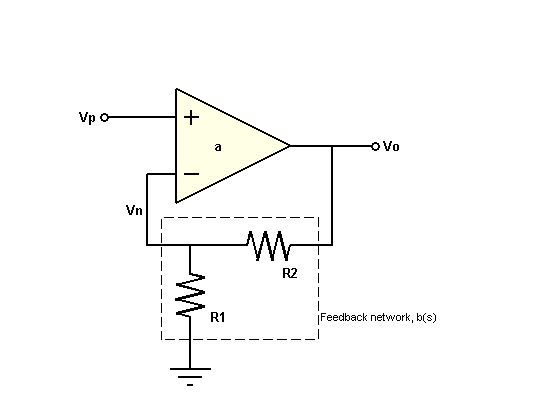The very small low-frequency sensitivity (about -80 dB) indicates a design whose closed-loop gain suffers minimally from open-loop gain variation. Such variation in a(s) is common due to manufacturing variability, temperature change, etc.

You can check the step response of A(s) using the STEPPLOT command:

stepplot(A) % Annotation opampdemo_annotate(2)Note that the use of feedback has greatly reduced the settling time (by about 98%). However, the step response now displays a large amount of ringing, indicating poor stability margin.

You can analyze the stability margin by plotting the loop gain, L(s), with the MARGIN command:

margin(L)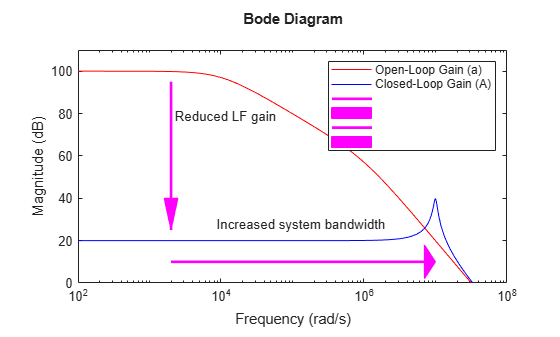The resulting plot indicates a phase margin of less than 6 degrees. You will need to compensate this amplifier in order to raise the phase margin to an acceptable level (generally 45 deg or more), thus reducing excessive overshoot and ringing.

A commonly used method of compensation in this type of circuit is "feedback lead compensation". This technique modifies b(s) by adding a capacitor, C, in parallel with the feedback resistor, R2.The capacitor value is chosen so as to introduce a phase lead to b(s) near the crossover frequency, thus increasing the amplifier's phase margin.

The new feedback transfer function is shown below.You can approximate a value for C by placing the zero of b(s) at the 0dB crossover frequency of L(s):

[Gm,Pm,Wcg,Wcp] = margin(L); C = 1/(R2*Wcp) 
C = 1.1139e-12 

To study the effect of C on the amplifier response, create an LTI model array of b(s) for several values of C around your initial guess:K = R1/(R1+R2); C = [1:.2:3]*1e-12; for n = 1:length(C) b_array(:,:,n) = tf([K*R2*C(n) K],[K*R2*C(n) 1]); end 

Now you can create LTI arrays for A(s) and L(s):

A_array = feedback(a,b_array); L_array = a*b_array; 

You can plot the step response of all models in the LTI array, A_array(s), together with A(s) using the STEPPLOT command:

stepplot(A,'b:',A_array,'b',[0:.005:1]*1.5e-6); title('Closed-Loop Step Response (Compensated)'); % Plot Annotations opampdemo_annotate(3)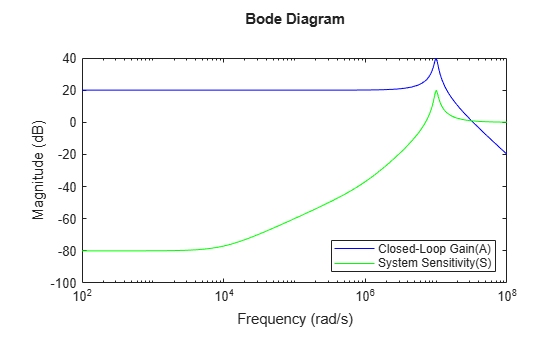The phase margins for our loop gain array, L_array(s), are found using the MARGIN command:

[Gm,Pm,Wcg,Wcp] = margin(L_array); 

The phase margins may now be plotted as a function of C.

plot(C*1e12,Pm,'g'); ax = gca; xlim([0.8 3.6]); ylim([45 60]); ax.Box = 'on'; xlabel('Compensation Capacitor, C (pF)'); ylabel('Phase Margin (deg)') % Plot Annotations opampdemo_annotate(4)A maximum phase margin of 58 deg is obtained when C=2pF (2e-12).

The model corresponding to C=2pF is the sixth model in the LTI array, b_array(s). You can plot the step response of the closed- loop system for this model by selecting index 6 of the LTI array A_array(s):

A_comp = A_array(:,:,6); stepplot(A,'b:',A_comp,'b') legend('Uncompensated (0 pF)','Compensated (2 pF)')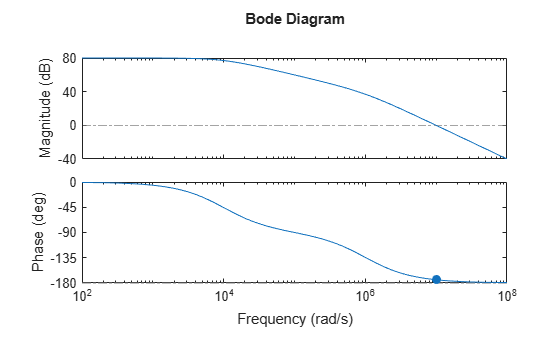Note that the settling time has been further reduced (by an additional 85%).

We can overlay the frequency-response of all three models (open-loop, closed-loop, compensated closed-loop) using the BODE command:

bodeplot(a,'r',A,'b:',A_comp,'b') legend('a(s)','A(s)','A\_comp(s)');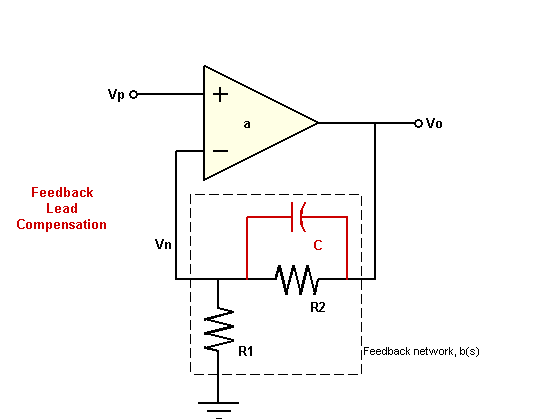Note how the addition of the compensation capacitor has eliminated peaking in the closed-loop gain and also greatly extended the phase margin.

### Summary

A brief summary of the choice of component values in the design of this non-inverting feedback amplifier circuit:

• Final component values: R1 = 10 kOhm, R2 = 90 kOhm, C = 2 pF.

• A resistive feedback network (R1,R2) was selected to yield a broadband amplifier gain of 10 (20 dB).

• Feedback lead compensation was used to tune the loop gain near the crossover frequency. The value for the compensation capacitor, C, was optimized to provide a maximum phase margin of about 58 degrees.